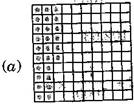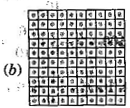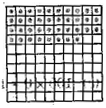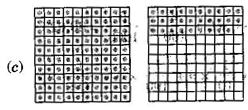1. /
2. CBSE
3. /
4. Class 06
5. /
6. Mathematics
7. /
8. NCERT Solutions for Class...

# NCERT Solutions for Class 6 Maths Exercise 8.2

NCERT solutions for Maths Decimals## NCERT Solutions for Class 6 Maths Decimals

###### Question 1.Complete the table with the help of these boxes and use decimals to write the number:Ones Tenths Hundreths Numbers {tex}\left( a \right){/tex} {tex}\left( b \right){/tex} {tex}\left( c \right){/tex}

 Ones Tenths Hundreths Numbers {tex}\left( a \right){/tex} 0 2 6 0.26 {tex}\left( b \right){/tex} 1 3 8 1.38 {tex}\left( c \right){/tex} 1 2 8 1.28

###### Question 2.Write the numbers given in the following place value table in decimal form:
 Hundreds100 Tens10 Ones1 Tenths{tex}\frac{1}{{10}}{/tex} Hundreths{tex}\frac{1}{{100}}{/tex} Thousandths{tex}\frac{1}{{1000}}{/tex} {tex}\left( a \right){/tex}{tex}\left( b \right){/tex}{tex}\left( c \right){/tex}{tex}\left( d \right){/tex}{tex}\left( e \right){/tex} 01020 00311 32012 26092 53204 00521

(a) 0 x 100 + 0 x 10 + 3 x 1 + 2 x {tex}\frac{1}{{10}}{/tex} + 5 x {tex}\frac{1}{{100}}{/tex} + 0 x {tex}\frac{1}{{1000}}{/tex}

= 0 + 0 + 3 + 0.2 + 0.05 + 0 = 3.25

(b) 1 x 100 + 0 x 10 + 2 x 1 + 6 x {tex}\frac{1}{{10}}{/tex} + 3 x {tex}\frac{1}{{100}}{/tex} + 0 x {tex}\frac{1}{{1000}}{/tex}

= 1 + 0 + 2 + 0.6 + 0.03 + 0 = 102.63

(c) 0 x 100 + 3 x 10 + 0 x 1 + 0 x {tex}\frac{1}{{10}}{/tex} + 2 x {tex}\frac{1}{{100}}{/tex} + 5 x {tex}\frac{1}{{1000}}{/tex}

= 0 + 30 + 0 + 0 + 0.02 + 0.005 = 30.025

(d) 2 x 100 + 1 x 10 + 1 x 1 + 9 x {tex}\frac{1}{{10}}{/tex} + 0 x {tex}\frac{1}{{100}}{/tex} + 2 x {tex}\frac{1}{{1000}}{/tex}

= 200 + 10 + 1 + 0.9 + 0 + 0.002 = 211.902

(e) 0 x 100 + 1 x 10 + 2 x 1 + 2 x {tex}\frac{1}{{10}}{/tex} + 4 x {tex}\frac{1}{{100}}{/tex} + 1 x {tex}\frac{1}{{1000}}{/tex}

0 + 10 + 2 + 0.2 + 0.04 + 0.001 = 12.241

NCERT Solutions for Class 6 Maths Exercise 8.2

###### Question 3.Write the following decimals in the place value table:

(a) 0.29

(b) 2.08

(c) 19.60

(d) 148.32

(e) 200.812

 Numbers Hundreds Tens Ones Tenths Hundreths Thousandths 100 10 1 {tex}\frac{1}{{10}}{/tex} {tex}\frac{1}{{100}}{/tex} {tex}\frac{1}{{1000}}{/tex} (a) 0.29 0 0 0 2 9 0 (b) 2.08 0 0 2 0 8 0 (c) 19.60 0 1 9 6 0 0 (d) 148.32 1 4 8 3 2 0 (e) 200.812 2 0 0 8 1 2

###### Question 4.Write each of the following as decimals:

(a) {tex}20 + 9 + \frac{4}{{10}} + \frac{1}{{100}}{/tex}

(b) {tex}137 + \frac{5}{{100}}{/tex}

(c) {tex}\frac{7}{{10}} + \frac{6}{{100}} + \frac{4}{{1000}}{/tex}

(d) {tex}23 + \frac{2}{{10}} + \frac{6}{{1000}}{/tex}

(e) {tex}700 + 20 + 5 + \frac{9}{{100}}{/tex}

(a) 20 + 9 + 0.4 + 0.01 = 29.41

(b) 137 + 0.05 = 137.05

(c) 0.7 + 0.06 + 0.004 = 0.764

(d) 23 + 0.2 + 0.006 = 23.206

(e) 700 + 20 + 5 + 0.09 = 725.09

NCERT Solutions for Class 6 Maths Exercise 8.2

###### Question 5.Write each of the following decimals in words:

(a) 0.03

(b) 1.20

(c) 108.56

(d) 10.07

(e) 0.032

(f) 5.008

(a) Zero point zero three

(b) One point two zero

(c) One hundred and eight point five six

(d) Ten point zero seven

(e) Zero point zero three two

(F) Five point zero zero eight

###### Question 6.Between which two numbers in tenths place on the number line does each of the given number lie?

(a) 0.06

(b) 0.45

(c) 0.19

(d) 0.66

(e) 0.92

(f) 0.57

All the numbers lie between 0 and 1.

(a) 0.06 is nearer to 0.1.

(b) 0.45 is nearer to 0.5.

(c) 0.19 is nearer to 0.2.

(d) 0.66 is nearer to 0.7.

(e) 0.92 is nearer to 0.9.

(f) 0.57 is nearer to 0.6.

NCERT Solutions for Class 6 Maths Exercise 8.2

###### Question 7.Write as fractions in lowest terms:

(a) 0.60

(b) 0.05

(c) 0.75

(d) 0.18

(e) 0.25

(f) 0.125

(g) 0.066

(a) 0.60 = {tex}\frac{60}{100}=\frac{3}{5}{/tex}

(b) 0.05 = {tex}\frac{5}{100}=\frac{1}{20}{/tex}

(c) 0.75 = {tex}\frac{75}{100}=\frac{3}{4}{/tex}

(d) 0.18 = {tex}\frac{18}{100}=\frac{9}{50}{/tex}

(e) 0.25 = {tex}\frac{25}{100}=\frac{1}{4}{/tex}

(f) 0.125 = {tex}\frac{125}{1000}=\frac{1}{8}{/tex}

(g) 0.066 = {tex}\frac{66}{1000}=\frac{33}{500}{/tex}

## NCERT Solutions for Class 6 Maths Exercise 8.2

NCERT Solutions Class 6 Maths PDF (Download) Free from myCBSEguide app and myCBSEguide website. Ncert solution class 6 Maths includes text book solutions from Class 6 Maths Book . NCERT Solutions for CBSE Class 6 Maths have total 14 chapters. 6 Maths NCERT Solutions in PDF for free Download on our website. Ncert Maths class 6 solutions PDF and Maths ncert class 6 PDF solutions with latest modifications and as per the latest CBSE syllabus are only available in myCBSEguide.

## CBSE app for Students

To download NCERT Solutions for Class 6 Maths, Social Science Computer Science, Home Science, Hindi English, Maths Science do check myCBSEguide app or website. myCBSEguide provides sample papers with solution, test papers for chapter-wise practice, NCERT solutions, NCERT Exemplar solutions, quick revision notes for ready reference, CBSE guess papers and CBSE important question papers. Sample Paper all are made available through the best app for CBSE students and myCBSEguide website.Test Generator

Create question paper PDF and online tests with your own name & logo in minutes.myCBSEguide

Question Bank, Mock Tests, Exam Papers, NCERT Solutions, Sample Papers, Notes

### 1 thought on “NCERT Solutions for Class 6 Maths Exercise 8.2”

1. It’s very useful to learn thank you so much for giving the solution because of this I can study by myself